# Common Core: High School - Geometry : Geometric Methods to Solve Design Problems: CCSS.Math.Content.HSG-MG.A.3

## Example Questions

### Example Question #1 : Geometric Methods To Solve Design Problems: Ccss.Math.Content.Hsg Mg.A.3

Suppose you have a 12ft x 12ft area that you want to lay down tile for.  Your tiles are 2ft x 2ft each.  How many tiles do you need to perform this task?

36 tiles

4 tiles

64 tiles

144 tiles

36 tiles

Explanation:

When it comes to word problems such as these, always begin by drawing an illustration.  Below is a picture of our 12 x 12 area we need to fill with tiles and one 2 x 2 tile that we are going to use to fill this area.There are two methods that we can use to solve this problem:

Method 1:

First we need to ask, how many tiles are needed to line the width of this 12 x 12 area.  So if we have tiles that are 2 ft each we need to divide 12 by 2 to figure out how many tiles we need to line the width.And so we need 6 tiles to line the width.

No we need to ask, how many tiles are needed to line the height of this 12 x 12 area.  So if we have tiles that are 2 ft each we need to divide 12 by 2 to figure out how many tiles we need to line the height.And so we need 6 tiles to line the width.

Now we need to find the total amount of tiles needed to fill the entire 12 x 12 space.  We can solve for this just like we solve for area.  We will multiply the amount of tiles across the width by the amount of tiles lining the height.  This gives us an “area” in terms of the amount of tiles needed to fill the space.And so we need 36 tiles to fill the space

Method 2:

We begin by finding the area of the entire 12 x 12 space.  We use the fact thatAnd so our area of the entire space is.  Now we need to find the area of each individual tile.And so the area of each individual tile is.  To find the total amount of tiles needed we need to find the amount ofsections in the totalarea.  To do this we divide:### Example Question #1 : Geometric Methods To Solve Design Problems: Ccss.Math.Content.Hsg Mg.A.3

You have a barrel (cylinder) that is 1ft in radius and 3 ft in height.  There was a thunderstorm the night before and you have found that the barrel is full of water.  How much water is in the barrel?  Round to the second decimal

172.93L

266.74L

156.01L

9.42L

266.74L

### Example Question #1 : Geometric Methods To Solve Design Problems: Ccss.Math.Content.Hsg Mg.A.3

You have been collecting stamps for a couple years now.  You now think you have enough to decorate your right-angle triangular prism shaped jewelry box without leaving any open gaps.  The triangular faces of your jewelry box have a height of 5in and a base length of 3in.  The rectangular faces of your jewelry box have a height of 5in and a width of 7in.  You have stamps that are each 1in x 1in squares.  Do you have enough stamps?

No

Yes

There is not enough information

No

Explanation:

The goal of this problem is to find the surface area of the triangular prism and see if you have enough 1in x 1in stamps to fill the surface area without leaving any open gaps.  To find the surface area of the prism we know that there are two triangular faces, and three rectangular faces.  We will find the area of each of these and then their sum is the total surface area.

Area for each triangular face:Area for each rectangular face:So now we will sum 2 triangular faces and three rectangular faces for the total surface area.Since we want to find if we have enough stamps, we will divide the total surface area by the surface area of an individual stamp to find how many stamps we would need to cover the prism entirely.We only have 112 stamps, so we do not have enough stamps.

### Example Question #1 : Geometric Methods To Solve Design Problems: Ccss.Math.Content.Hsg Mg.A.3

True or False: We want to find the amount of sod squares to lay within a fenced in area.  This is a volume question.

True

False

False

Explanation:

This is an area problem.  For a fenced in, flat area, we would only consider the height and width.  To be a volume problem we would need to have a 3-dimensional space to fill, one with width, length, and height.

### Example Question #1 : Geometric Methods To Solve Design Problems: Ccss.Math.Content.Hsg Mg.A.3

True or false: You want to find the radius of your water bottle.  Assuming your water bottle is a cylinder and you know it holds 10oz of water, you are able to determine the radius.

False

True

False

Explanation:

The formula to find the volume of a cylinder is.  While we have the volume of the bottle, we do not have the height.  In order to work backwards to find the radius we would also need the height of the cylinder.

### Example Question #2 : Geometric Methods To Solve Design Problems: Ccss.Math.Content.Hsg Mg.A.3

You would like to construct an open face rectangular prism to hold some garden plants.  The prism is shown in the figure below.  If the entire prism will be filled with potting soil, how much potting soil will you need?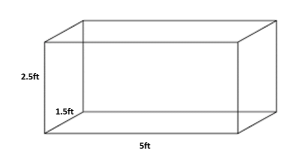9ft3

17.25ft3

18.75ft3

12.5ft3

18.75ft3

Explanation:

We need to understand that this is a volume question.  We are trying to find the total volume of potting soil needed to fill this rectangular prism.  The formula to find the volume of a rectangular prism is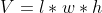.  The length of the prism is 5ft, the width of the prism is 1.5ft, and the height of the prism is 2.5. We will plug these into our formula to solve this problem.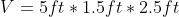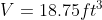### Example Question #31 : Modeling With Geometry

You need to build a diagonal support for the bleachers at the local sports field.  The support needs to reach from the ground to the top of the bleacher.  How the support should look is highlighted in blue below.  The bleacher wall is 10 feet high and perpendicular to the ground.  The owner would like the support to only stick out 3 feet from the bleacher at the bottom.  What is the length of the support you need to build?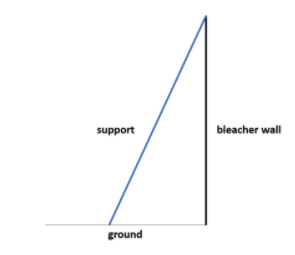109 ft

11.32 ft

10.44 ft

20 ft

10.44 ft

Explanation:

It is important to recognize that the bleacher, the ground, and the support form a right triangle with the right angle formed by the intersection of the bleacher wall and the ground.  We know the bottom of the support should only be 3ft from the bleacher wall on the ground and the bleacher wall is 10ft high.  We will use the Pythagorean Theorem to solve for the length of the support, which is the hypotenuse of this right triangle.  Our base of the triangle is 3 feet and the leg is 10 feet.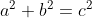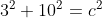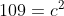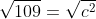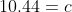And so we need a support of 10.44 feet long.

### Example Question #1 : Geometric Methods To Solve Design Problems: Ccss.Math.Content.Hsg Mg.A.3

You would like to fill a hollow globe with candy for your students.  Since the globe opens upwards at the center, you can only fill the globe halfway.  We cannot overfill the globe.  If the globe has a radius of 15cm and each spherical candy takes up 2cm3 of space, how many candies do you need to fill the globe halfway?

3,532 candies

14,130 candies

7,065 candies

471 candies

3,532 candies

Explanation:

This is a volume question, asking for the volume of a sphere.  There is a twist; we only want half of the volume of the globe.  We will start by using the volume formula for a sphere,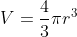.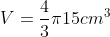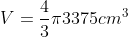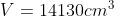But we want half of the total volume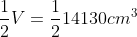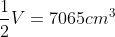So we know the volume of half of the globe is 7,065cm3.  Since each candy is 2cm3, we divide the volume of the space we need to take up by this amount to find the number of individual candies we need.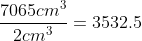We cannot have half of a candy, however, and we do not want to overfill the globe.  So we round down to fill the globe with 3,532 candies.

### All Common Core: High School - Geometry Resources